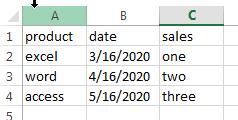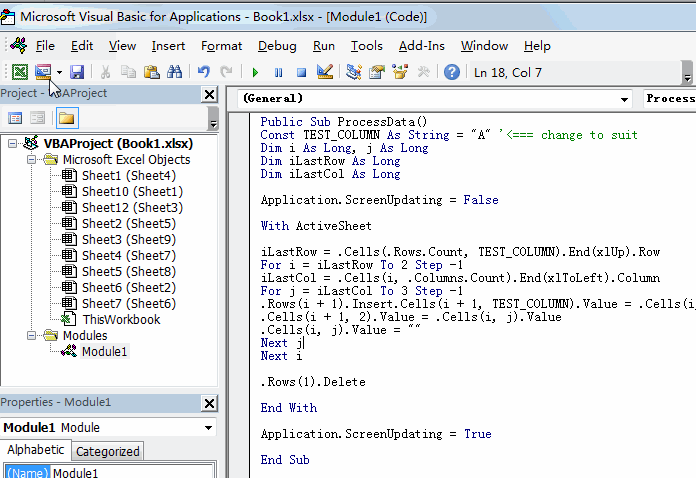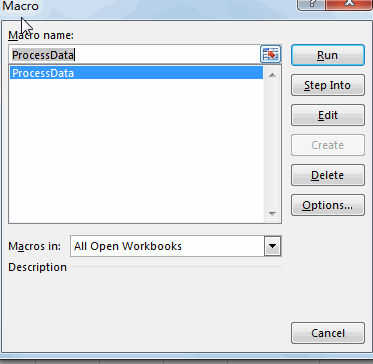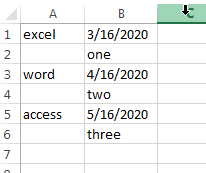# How to Transpose Cross Tab to Flat Table in Excel

This post will guide you how to convert cross table to list in Excel 2013/2016. How do I transpose cross table to flat table with VBA Macro in Excel.

CrossTab (cross table) is a two-dimensional table with a horizontal header row and a vertical header row. Assuming that you have a list of data in range A1:C5, and you want to convert this cross table or two dimensional table to a list or flat table. How to do it. You can  use VBA Macro to achieve the result quickly. Just do the following steps:#1 open your excel workbook and then click on “Visual Basic” command under DEVELOPER Tab, or just press “ALT+F11” shortcut.#2 then the “Visual Basic Editor” window will appear.

#3 click “Insert” ->”Module” to create a new module.#4 paste the below VBA code  into the code window. Then clicking “Save” button.```Public Sub ProcessData()
Const TEST_COLUMN As String = "A"
Dim i As Long, j As Long
Dim iLastRow As Long
Dim iLastCol As Long
Application.ScreenUpdating = False
With ActiveSheet
iLastRow = .Cells(.Rows.Count, TEST_COLUMN).End(xlUp).Row
For i = iLastRow To 2 Step -1
iLastCol = .Cells(i, .Columns.Count).End(xlToLeft).Column
For j = iLastCol To 3 Step -1
.Rows(i + 1).Insert
.Cells(i + 1, 2).Value = .Cells(i, j).Value
.Cells(i, j).Value = ""
Next j
Next i
.Rows(1).Delete
End With
Application.ScreenUpdating = True
End Sub```

#5 back to the current worksheet, then run the above excel macro. Click Run button.#6 Let’s see the last result:### You might also like:

Sidebar